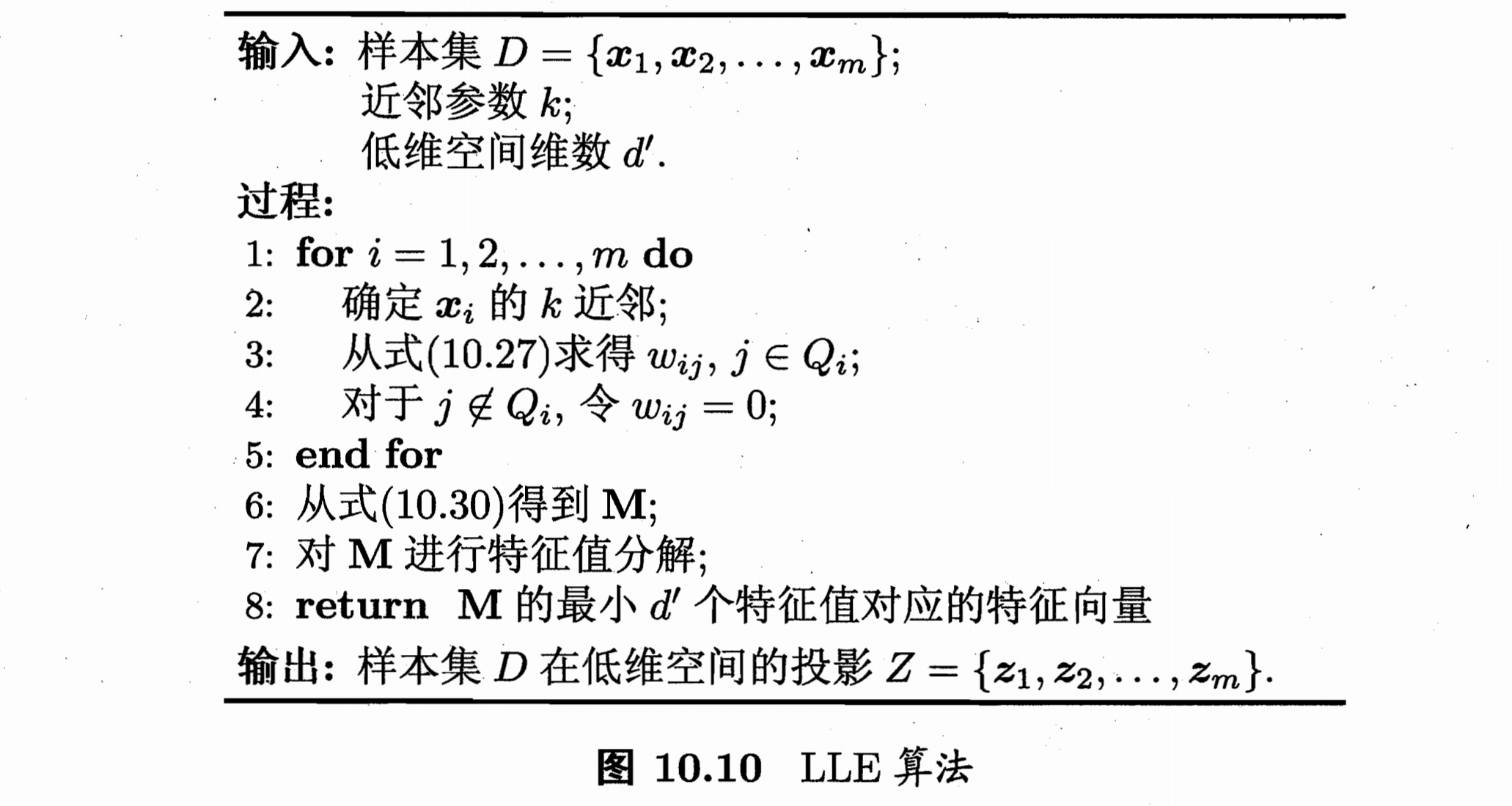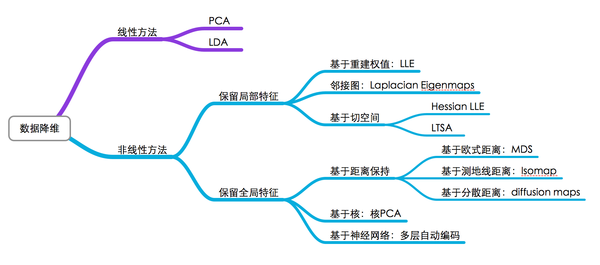## k近邻学习

k近邻学习是一种常用的监督学习方法, 工作的机制非常简单,对于每个给定的测试样本, 基于某种距离找出训练集中与其最相近的k个训练样本, 然后基于这些邻居的信息来预测该测试样本. 在分类任务中,可以采取投票法, 在回归任务中可以采取平均法, 更复杂一些也可以采取各种加权方式.

k近邻学习中两个重要的点:

• k的选取
• 距离的定义

## 主成分分析

PCA分析属于一种正交变换，即，将原始特征做正交变换，获得的每个特征都是原特征的线性组合，然后从中选出部分特征，作为变换的输出。

## 流形学习

### 等度量映射 IsomapIsomap算法

• 指定近邻点个数$k$, 连接最近的$k$个点作为近邻点
• 制定距离阈值$\varepsilon$, 距离小于该阈值的点作为近邻点

### 局部线性嵌入 LLE

LLE希望这个性质能在低维空间中得以保持. 那么对于每个$x_i$, LLE会根据近邻样本计算出线性重构的系数$w_i$ (拉格朗日乘子法).LLE算法

## 度量学习

### 近邻成分分析 NCA## 习题及参考答案

10.1 编程实现k近邻分类器，　在西瓜数据集3.0a上比较其分类边界与决策树分类边界的异同．

10.2 令$err$, $err*$分别表示最近邻分类器与贝叶斯最优分类器的期望错误率, 试证明:

10.3 在对高维数据降维之前应先进行”中心化”, 常见的是将协方差矩阵$XX^T$转为$XHH^TX^T$,其中$H=I-\frac{1}{m}11^T$,试分析其效果
(题目似乎有些问题, 1矩阵应该一个就够了即$11^T$应为$1$)

10.4 在实践中, 协方差矩阵$XX^T$的特征值分解常用中心化后的样本矩阵$X$的奇异值分解代替, 试述其原因.

10.5 降维中涉及的投影矩阵通常要求是正交的,试述正交,非正交投影矩阵用于降维的优缺点.

10.6 使用MATLAB中的PCA函数对Yale人脸数据集进行降维,并观察前20个特征向量所对应的图像.

10.7 试述核化线性降维和流形学习之间的联系和优缺点.

10.8 $k$近邻图和$\varepsilon$近邻图存在的短路和断路问题会给Isomap造成困扰,试设计一个方法缓解该问题.

• 把两种构图方法结合一下, 当距离小于$\varepsilon$范围内有近邻点时设定其最近的点为近邻点.
• 当发生短路时，在近邻范围内启发式人为增加样本（例如增加指定点和其最近邻的中点）,当然这么做会改变样本分布, 当数据很稀疏时不能这么玩.

10.9 试设计一个方法为新样本找到LLE降维后的低维坐标

10.10 试述如何确保度量学习产生的距离能满足距离度量的四条基本性质.

• 非负性 $d\ge 0$
• 同一性 $d_{ij}=0$当且仅当$i=j$
• 对称性 $d_{ij}=d_{ji}$
• 直递性 $d_{ij}\le d_{ik}+d_{kj}$